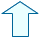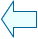ACTIVITIES WITH LINEAR FUNCTIONS Analysis

The gradient of a straight line depends on the coordinates of the points it passes through.

y = m x

 Give a value for m using decimals or fractions like 5/7 or -1/2 and then press the Enter key. If you get it right the function is expressed in orange but if you are wrong it is expressed in red.

1.- Click on the Start button (init) to change the points to 0. Choose the number that corresponds to the gradient of the orange straight line by looking carefully at the coordinates of the green point on the line.

 After each correct answer click on the animate button to get a new line.
2.- Repeat the process until you get 10 points.

We need to find a method that allows us to guess the gradient of a linear function by reading its graph.

y = m x

 This is similar to the previous activity but this time you have to use the zoom to find the green point before writing in the gradient m. Remember to click on the 'init' button first.

3.- Find the gradient of the orange line. First locate the green point and look at its coordinates.

 If you click the left hand button of the mouse on the point the coordinates of the point will appear on the screen.

4.- Repeat the process until you get 10 points.

5.- In your notebook explain how we can obtain the gradient of a line that passes through a given point.

Now we need to know how to estimate the  gradient of any straight line.

y = m x

6.- Estimate the gradient of the orange line.

 Use the left hand button of the mouse to get the coordinates of any point you click on. Remember to click on the 'init' button first.
 After each correct answer click on the animate button to get a new straight line.

7.- Repeat the process until you get 10 points.

4. THE ANGLE BETWEEN A STRAIGHT LINE AND THE SEMI-AXIS OX.
The angle formed by a straight line and the semi-axis OX (positive coordinates along the x-axis) is connected to its gradient. The gradient of the line is the same as the tangent of this angle formed by the straight line and the semi-axis OX.

y = m x

 Drag the green button with the mouse.

8.- Look carefully at the values given for the angle formed with different straight lines in each quadrant.

9.- Write down the gradients of the straight lines for the following angles:

 angle gradient 0º 0º-45º 45º 45º-90º 90º 90º-135º 135º 135º-180º 180ºJuan Madrigal MugaSpanish Ministry of Education. Year 2001